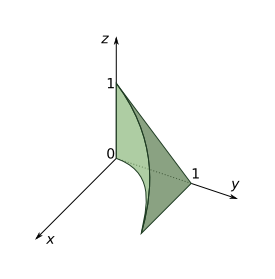Triple Integrals

(requires JavaScript)

1. Integrate $\underset{B}{\iiint }xy{z}^{2}\phantom{\rule{0.2em}{0ex}}dV$ over the box $B=\left[0,1\right]×\left[-1,2\right]×\left[0,3\right]$ first with respect to $z$, then $x$, and then $y$.
$\frac{27}{4}$
2. Evaluate ${\int }_{0}^{3}{\int }_{0}^{1}{\int }_{0}^{\sqrt{1-{z}^{2}}}z{e}^{y}\phantom{\rule{0.2em}{0ex}}dx\phantom{\rule{0.2em}{0ex}}dz\phantom{\rule{0.2em}{0ex}}dy$.
$\frac{1}{3}\left({e}^{3}-1\right)$
3. Evaluate the triple integral $\underset{E}{\iiint }yz\mathrm{cos}\left({x}^{5}\right)\phantom{\rule{0.2em}{0ex}}dV$ where

$E=\left\{\left(x,y,z\right)|0\le x\le 1,\phantom{\rule{0.5em}{0ex}}0\le y\le x,\phantom{\rule{0.5em}{0ex}}x\le z\le 2x\right\}$.

4. Evaluate the triple integral $\underset{E}{\iiint }y\phantom{\rule{0.2em}{0ex}}dV$ where $E$ is bounded by the planes $x=0$, $y=0$, $z=0$, and $2x+2y+z=4$.

$\frac{4}{3}$
5. Evaluate the triple integral $\underset{E}{\iiint }x\phantom{\rule{0.2em}{0ex}}dV$ where $E$ is bounded by the paraboloid $x=4{y}^{2}+4{z}^{2}$ and the plane $x=4$.

$\frac{16\pi }{3}$
6. Use a triple integral to find the volume of the solid bounded by the cylinder ${x}^{2}+{y}^{2}=9$ and the planes $y+z=5$ and $z=1$.
$36\pi$
7. Express the integral $\underset{E}{\iiint }f\left(x,y,z\right)\phantom{\rule{0.2em}{0ex}}dV$ as an iterated integral in $6$ different ways if $E$ is the solid bounded by the surfaces $z=0$, $x=0$, $y=2$, and $z=y-2x$.
8. The figure shows the region of integration for the integral ${\int }_{0}^{1}{\int }_{\sqrt{x}}^{1}{\int }_{0}^{1-y}f\left(x,y,z\right)\phantom{\rule{0.2em}{0ex}}dz\phantom{\rule{0.2em}{0ex}}dy\phantom{\rule{0.2em}{0ex}}dx$. Rewrite this integral as an equivalent iterated integral in the $5$ other orders.9. Write $5$ other iterated integrals that are equal to the integral ${\int }_{0}^{1}{\int }_{y}^{1}{\int }_{0}^{y}f\left(x,y,z\right)\phantom{\rule{0.2em}{0ex}}dz\phantom{\rule{0.2em}{0ex}}dx\phantom{\rule{0.2em}{0ex}}dy$.
10. Find the average value of the function $f\left(x,y,z\right)={x}^{2}z+{y}^{2}z$ over the region enclosed by the paraboloid $z=1-{x}^{2}-{y}^{2}$ and the plane $z=0$.
11. Find the region $E$ for which the triple integral

$\underset{E}{\iiint }\left(1-{x}^{2}-2{y}^{2}-3{z}^{2}\right)\phantom{\rule{0.2em}{0ex}}dV$

is a maximum.

The region bounded by the ellipsoid ${x}^{2}+2{y}^{2}+3{z}^{2}=1$